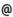## "Babes-Bolyai" University of Cluj-Napoca Faculty of Mathematics and Computer Science

 Selected topics of logical mathematics
 Code Semes-ter Hours: C+S+L Credits Type Section MA014 8 2+2+0 10 optional Informatică
 Teaching Staff in Charge
 Assoc.Prof. COVACI Rodica, Ph.D., rcovacimath.ubbcluj.ro
 Aims Determination of the connection between the speculative and the applied aspect of mathematical logic, due to computer science.
 Content 1. The algebra of propositions. Propositions. Propositional formulae. Logical implication and logical equivalence as relations. Lyndenbaum-Tarski algebra. Boole rings and algebras. 2. n-ary bivalent functions. Normal perfect forms. Synthesis problems. Applications. 3. Deductive theories. Interpretation and model. Non-contradiction and consistency. Completeness and categoricity. 4. Predicate logics.
 References 1. N. BOTH: Algebra logicii cu aplicatii, Ed. Dacia, Cluj-Napoca 1984. 2. N. BOTH: Capitole speciale de logica matematica, Lito, Univ Babes Bolyai Cluj-Napoca 1994. 3. P. NOVICOV: Elemente de logica matematica, Ed. Tehn., 1966. 4. Y.I. MANIN: A Course in Mathematical Logic, Springer-Verlag, New York 1977 5. S.G. KRANTZ: Logic and Proof Techniques for Computer Science, Birkhauser Boston 2002. 6. R. LIDL, G. PILZ: Applied Abstract Algebra, Springer-Verlag, Berlin, 1998.
 Assessment Two reports throughout the semester (each representing 20% of the final mark) and oral exam at the end of the semester (representing 60% of the final mark).